Get inspired by the success stories of our students in IIT JAM MS, ISI  MStat, CMI MSc DS.  Learn More

# Application of Cauchy Functional Equations | ISI MStat 2019 PSB Problem 4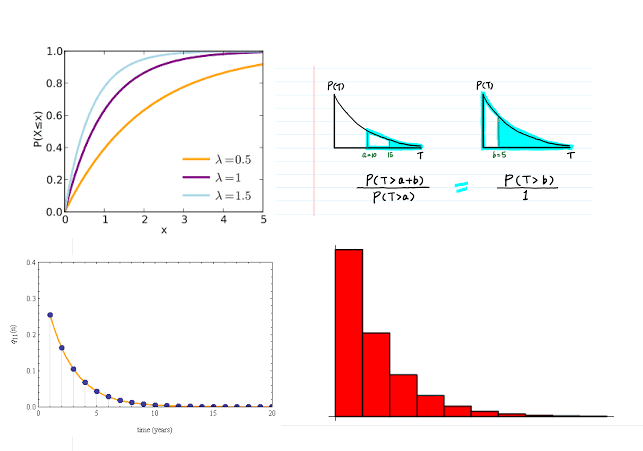This problem is a beautiful application of the probability theory and cauchy functional equations. This is from ISI MStat 2019 PSB problem 4.

## Problem - Application of Cauchy Functional Equations

Let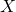and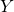be independent and identically distributed random variables with meanand taking values in {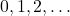}. Suppose, for all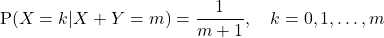Find the distribution ofin terms of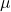.

### Prerequisites

• Conditional Probability
• Geometric Distribution
•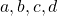are numbers such that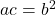&, thenare in geometric progression.

## Solution

Let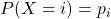where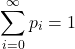. Now, let's calculate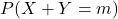.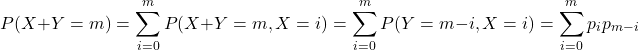.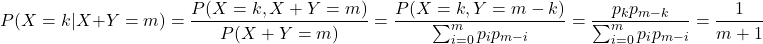.

Hence,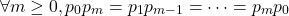.

Thus, we get the following set of equations.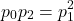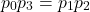Hence, by the thrid prerequisite,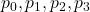are in geometric progression.

Observe that as a result we get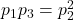. In the line there is waiting: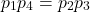. Thus, in the similar way we get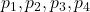are in geometric progression.

Hence, by induction, we will get that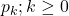form a geometric progression.

This is only possible if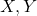~ Geom(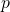). We need to findnow, but herecounts the number of failures, andis the probability of success.

So,.

### Challenge Problem

So, can you guess, what it will be its continous version?

It will be the exponential distribution. Prove it. But, what exactly is governing this exponential structure? What is the intuition behind it?

## The Underlying Mathematics and Intuition

Observe that the obtained condition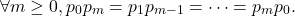can be written as follows

Find all such functionssuch that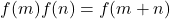and with the summation = 1 restriction property.

The only solution to this geometric progression structure. This is a variant of the Cauchy Functional Equation. For the continuous case, it will be exponential distribution.

Essentially, this is the functional equation that arises, if you march along to prove that the Geometric Random Variable is the only discrete distribution with the memoryless property.

Stay Tuned!

This problem is a beautiful application of the probability theory and cauchy functional equations. This is from ISI MStat 2019 PSB problem 4.

## Problem - Application of Cauchy Functional Equations

Letandbe independent and identically distributed random variables with meanand taking values in {}. Suppose, for allFind the distribution ofin terms of.

### Prerequisites

• Conditional Probability
• Geometric Distribution
•are numbers such that&, thenare in geometric progression.

## Solution

Letwhere. Now, let's calculate...

Hence,.

Thus, we get the following set of equations.Hence, by the thrid prerequisite,are in geometric progression.

Observe that as a result we get. In the line there is waiting:. Thus, in the similar way we getare in geometric progression.

Hence, by induction, we will get thatform a geometric progression.

This is only possible if~ Geom(). We need to findnow, but herecounts the number of failures, andis the probability of success.

So,.

### Challenge Problem

So, can you guess, what it will be its continous version?

It will be the exponential distribution. Prove it. But, what exactly is governing this exponential structure? What is the intuition behind it?

## The Underlying Mathematics and Intuition

Observe that the obtained conditioncan be written as follows

Find all such functionssuch thatand with the summation = 1 restriction property.

The only solution to this geometric progression structure. This is a variant of the Cauchy Functional Equation. For the continuous case, it will be exponential distribution.

Essentially, this is the functional equation that arises, if you march along to prove that the Geometric Random Variable is the only discrete distribution with the memoryless property.

Stay Tuned!

This site uses Akismet to reduce spam. Learn how your comment data is processed.

### Knowledge Partner